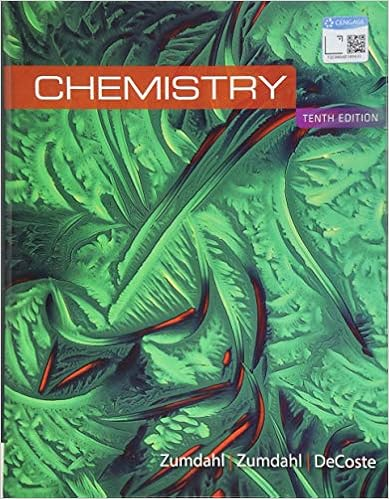# Nacl aq the 3 ca atoms in ca 3 po 4 2 on the right

• Homework Help
• 74
• 100% (1) 1 out of 1 people found this document helpful

This preview shows page 26 - 28 out of 74 pages.

##### We have textbook solutions for you!
The document you are viewing contains questions related to this textbook.The document you are viewing contains questions related to this textbook.
Chapter 17 / Exercise 35
Chemistry
ZumdahlExpert Verified
) + __NaCl(aq) The 3 Ca atoms in Ca3(PO4)2on the right require a coefficient of 3 in front of CaCl2on the left: 3CaCl2(aq) + __Na3PO4(aq) __Ca3(PO4)2(s) + __NaCl(aq) The 6 Cl atoms in 3CaCl2on the left require a coefficient of 6 in front of NaCl on the right: 3CaCl2(aq) + __Na3PO4(aq) __Ca3(PO4)2(s) + 6NaCl(aq) The 6 Na atoms in 6NaCl on the right require a coefficient of 2 in front of Na3PO4on the left: 3CaCl2(aq) + 2Na3PO4(aq) __Ca3(PO4)2(s) + 6NaCl(aq) There are now 2 P atoms on each side: 3CaCl2(aq) + 2Na3PO4(aq) Ca3(PO4)2(s) + 6NaCl(aq) 3.59Plan:The names must first be converted to chemical formulas. Balancing is a trial-and-error procedure. Balance one element at a time, placing coefficients where needed to have the same number of atoms of a particular element on each side of the equation. The smallest whole-number coefficients should be used. Remember that oxygen is diatomic. Solution:a) Aqueous solutions of lead(II) nitrate and potassium iodide are the reactants; solid lead(II) iodide and an aqueous solution of potassium nitrate are the products: __Pb(NO3)2(aq) + __KI(aq) __PbI2(s) + __KNO3(aq) There are 2 N atoms in Pb(NO3)2on the left so a coefficient of 2 is required in front of KNO3on the right: __Pb(NO3)2(aq) + __KI(aq) __PbI2(s) + 2KNO3(aq) The 2 K atoms in 2KNO3and the 2 I atoms in PbI2on the right require a coefficient of 2 in front of KI on the left: Pb(NO3)2(aq) + 2KI(aq) PbI2(s) + 2KNO3(aq) There are now 6 O atoms on each side: Pb(NO3)2(aq) + 2KI(aq) PbI2(s) + 2KNO3(aq) b) Liquid disilicon hexachloride and water are the reactants and solid silicon dioxide, hydrogen chloride gas and hydrogen gas are the products: __Si2Cl6(l) + __H2O(l) __SiO2(s) + __HCl(g) + __H2(g) The 2 Si atoms in Si2Cl6on the left require a coefficient of 2 in front of SiO2on the right: __Si2Cl6(l) + __H2O(l) 2SiO2(s) + __HCl(g) + __H2(g) The 6 Cl atoms in Si2Cl6on the left require a coefficient of 6 in front of HCl on the right: __Si2Cl6(l) + __H2O(l) 2SiO2(s) + 6HCl(g) + __H2(g)
##### We have textbook solutions for you!
The document you are viewing contains questions related to this textbook.The document you are viewing contains questions related to this textbook.
Chapter 17 / Exercise 35
Chemistry
ZumdahlExpert Verified
3-27The 4 O atoms in 2SiO2on the right require a coefficient of 4 in front of H2O on the left. __Si2Cl6(l) + 4H2O(l) 2SiO2(s) + 6HCl(g) + __H2(g) There are 8 H atoms in 4H2O on the left; there are 8 H atoms on the right (6 in 6HCl and 2 in H2): Si2Cl6(l) + 4H2O(l) 2SiO2(s) + 6HCl(g) + H2(g) c) Nitrogen dioxide and water are the reactants and an aqueous solution of nitric acid and nitrogen monoxide gas are the products: __NO2(g) + __H2O(l) __HNO3(aq) + __NO(g) Start with hydrogen it occurs in only one reactant and one product: The 2 H atoms in H2O on the left require a coefficient of 2 in front of HNO3on the right: __NO2(g) + __H2O(l) 2HNO3(aq) + __NO(g) The 3 N atoms on the right (2 in 2HNO3and 1 in NO) require a coefficient of 3 in front of NO2on the left; 3NO2(g) + __H2O(l) 2HNO3(aq) + __NO(g) There are now 7 O atoms on each side: 3NO2(g) + H2O(l) 2HNO3(aq) + NO(g) 3.60Plan: The names must first be converted to chemical formulas. Balancing is a trial-and-error procedure. Balance
•••